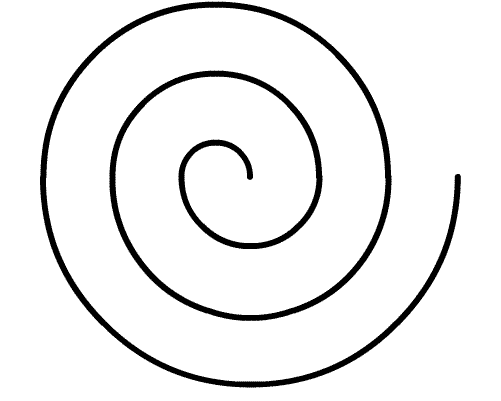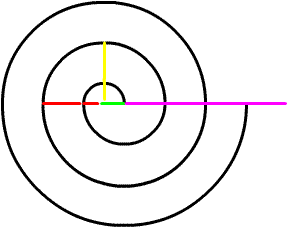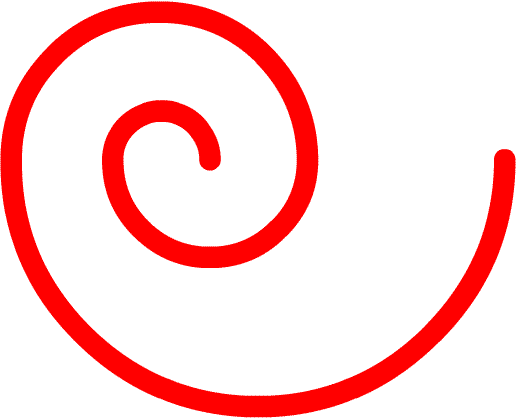Here is an involute with radius's of 1.25*(k*(2.22512/1.25))   Think of an involute as a string revolving around a pole. As the string becomes longer this is what is created.   We can use this to explain curves and to explain circular funtions such as the sine and cosine graph.   Read below than study the graphs that follow. Temporary Work _______ 20060806 As seen a changing circle or rather a changing chord can be expressed by an involute. (See Chord vs Circular Function) We can imagine a string unwinding around the circular function and that will lead to the discovery of all the values on the circular function. The rate of change is the key. The angles that are contained in the involute are proportional. That means that any involute representing the changing rate of the circle can be use. Pictured here is an involute used to solve a circle starting with a chord of 1.25 units. Here is the equations: 2.22512 = radius of first involute arc = radius of circle with chord 1.25 = distance covered at Pi radians 2.22512 * 2 = 4.45024 2.22512/1.25 = rate of change of involute per arc (at every Pi radians) 1.25 * 2.22512/1.25 = 2.22512 for remaining arcs... 1.25 * (2*( 2.22512/1.25 = 2.22512) = 4.45024 1.25 * (3*( 2.22512/1.25 = 2.22512) = 6.67536 It is important to note that this involute was drawn by geometric construction. Reference or construction is “Technical Drawing” Tenth Edition, Giesecke page 142 This common to draw an involute of a line was slightly modified to fit the rate of change of 2.22512/1.25 . The center of the 2.22512 radius circle was used as the line the involute was constructed around. This is so the unwrapping of the “string” would start at the end of the 1.25 chord. It is difficult to explain in words, but by studying the diagrams it is easily seen. _____________________________________________________________________________ Recalling the equation 1/(1.25/4) = 3.2... Now there is an equation for a logarithmic spiral. If the 1.25 chord was revolved and increased to maintain a circular arc. This logarithmic spiral can be drawn by connected every value of the involute by 2. So maybe we can find a simpler equation for both the involute and spiral. 3.2 + 1.25 = 4.45 4.45/2 = 2.22512 also the radius What is the application? Most importantly it describes circular functions such as the sine and cosine. Also it is its own coordinate system. Imagine how curves could be described only using elementary mathematics. But these are yet to be found! In this graph the small red line plus the length of the horizontal green line at the center is the radius 2.22512 respectively . This involute is based on a change of 2.22512 per Pi radians. Also pay attention to the two red lines combined. This is the diameter of the circle we solved for. It has a length of 3.2 units. That is taken from the end of the green line. Add the green line and you get the diameter of 4.45 units. If we were to base our shape on the diameter we would have drawn a logarithmic spiral.This shows the logarithmic spiral drawn drawn over the involute. Recall the equation 1/(1.25/4) = 3.2. This is very valuable information when solving for circular funtion. It would also be a challenge for the curious mathematicain to try and find the coordinate system that is created. Also we have found similarites between involutes and logarithmic spirals*Note do not confuse a logarithmic spiral with the Spiral of Archimedes. Admittedly these spirals can be confusing. A good source for a basic definition is www.wikipedia.org .... May the Creative Force be with You!